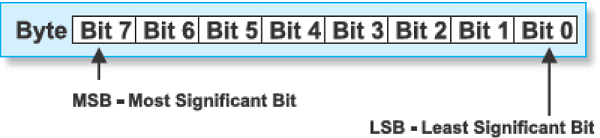# Arduino - Random Numbers

To generate random numbers, you can use Arduino random number functions. We have two functions −

• randomSeed(seed)
• random()

## randomSeed (seed)

The function randomSeed(seed) resets Arduino’s pseudorandom number generator. Although the distribution of the numbers returned by random() is essentially random, the sequence is predictable. You should reset the generator to some random value. If you have an unconnected analog pin, it might pick up random noise from the surrounding environment. These may be radio waves, cosmic rays, electromagnetic interference from cell phones, fluorescent lights and so on.

### Example

```randomSeed(analogRead(5)); // randomize using noise from analog pin 5
```

## random( )

The random function generates pseudo-random numbers. Following is the syntax.

### random( ) Statements Syntax

```long random(max) // it generate random numbers from 0 to max
long random(min, max) // it generate random numbers from min to max
```

### Example

```long randNumber;

void setup() {
Serial.begin(9600);
// noise will cause the call to randomSeed() to generate
// different seed numbers each time the sketch runs.
// randomSeed() will then shuffle the random function.
}

void loop() {
// print a random number from 0 to 299
Serial.print("random1=");
randNumber = random(300);
Serial.println(randNumber); // print a random number from 0to 299
Serial.print("random2=");
randNumber = random(10, 20);// print a random number from 10 to 19
Serial.println (randNumber);
delay(50);
}
```

Let us now refresh our knowledge on some of the basic concepts such as bits and bytes.

## Bits

A bit is just a binary digit.

• The binary system uses two digits, 0 and 1.

• Similar to the decimal number system, in which digits of a number do not have the same value, the ‘significance’ of a bit depends on its position in the binary number. For example, digits in the decimal number 666 are the same, but have different values.## Bytes

A byte consists of eight bits.

• If a bit is a digit, it is logical that bytes represent numbers.

• All mathematical operations can be performed upon them.

• The digits in a byte do not have the same significance either.

• The leftmost bit has the greatest value called the Most Significant Bit (MSB).

• The rightmost bit has the least value and is therefore, called the Least Significant Bit (LSB).

• Since eight zeros and ones of one byte can be combined in 256 different ways, the largest decimal number that can be represented by one byte is 255 (one combination represents a zero).